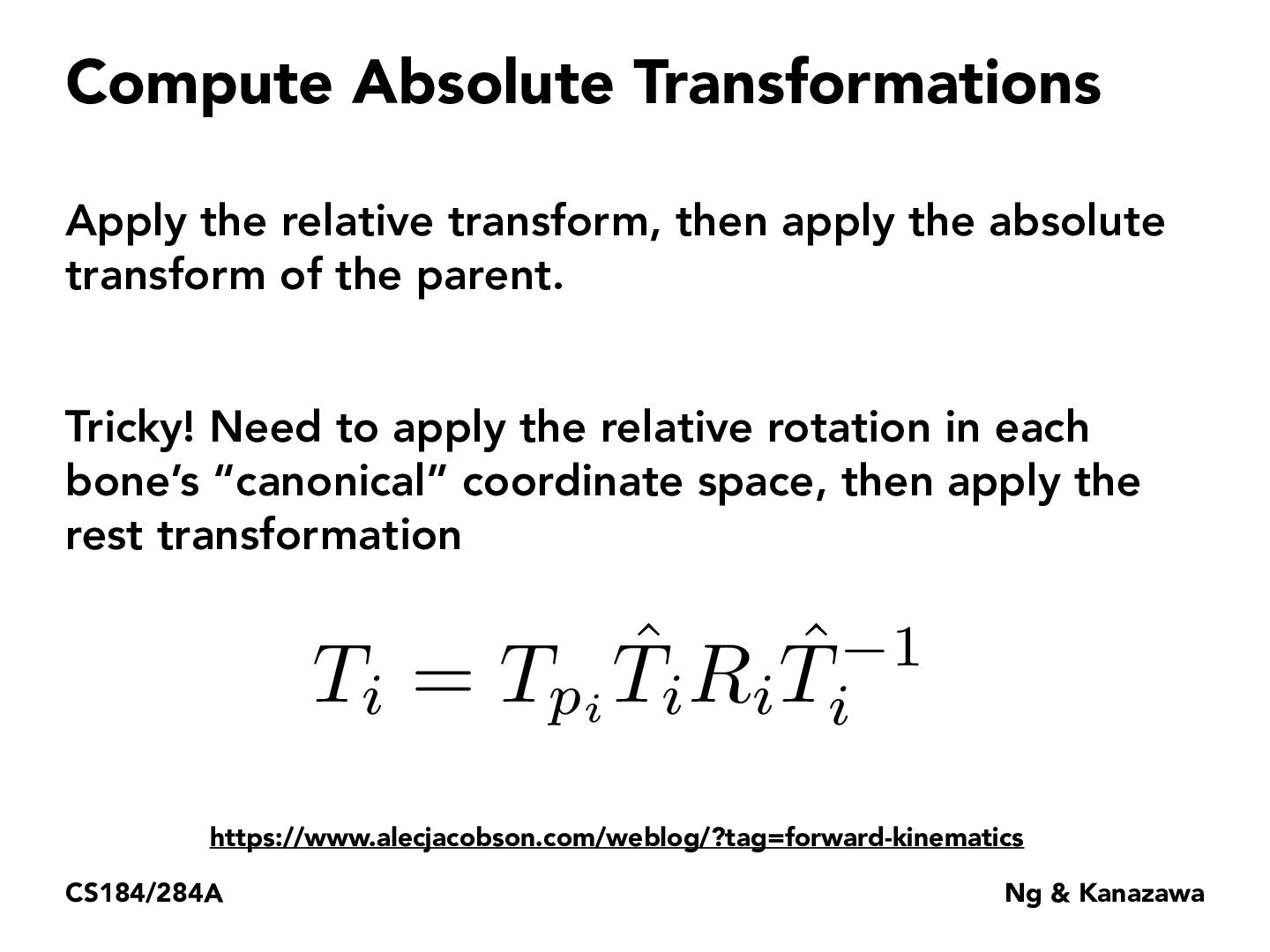Lecture 19: Introduction to Character Animations (12)herojelly

This is similar to the transforms we implemented in hw1, where we had to rotate an object, we had to move it to the origin, rotate it, and then move the object back to its original location.pixelled

$T_i$ represents the transformation from bone space to model space. Since in matrix form transformations are applied from right to left, we first apply the inverse $T_i$ to transfrom into bone space, then apply rotations, and transform back into model space and apply the rest $T_{p_i}$.XTLiuuu

[deleted]XTLiuuu

Can we apply the absolute transform before the relative transform?

You must be enrolled in the course to comment